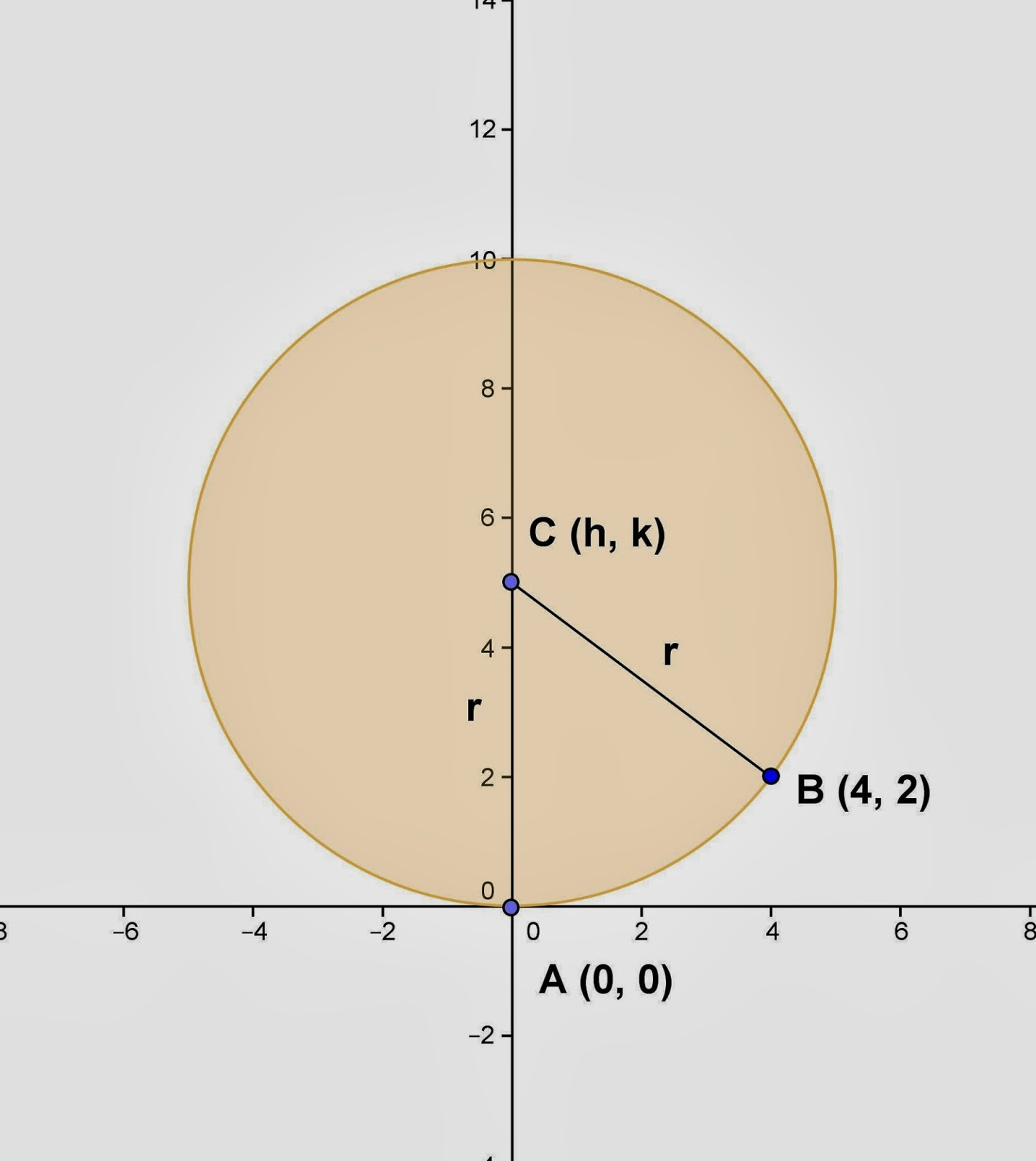## Monday, January 27, 2014

### Finding Equation - Circle, 5

Category: Analytic Geometry, Plane Geometry

"Published in Newark, California, USA"

Find the equation of a circle whose center on the y-axis, and passes through the origin and the point (4, 2).

Solution:

To illustrate the problem, it is better to draw the figure as followsPhoto by Math Principles in Everyday Life

In this problem, the center of a circle is unknown and we need to solve it. Since the two points of a circle are given, then we can solve for the coordinates of the center of a circle by using the distance of two points formula as follows

Since the given figure is a circle, then it follows that their distances from the center are equal as follows

Square on both sides of the equation, we have

Since the center of a circle is located along the y-axis, then it follows that h = 0.

Hence, the center of a circle is C (0, 5). The radius of a circle can be solved by using the distance of two points formula as follows

Therefore, the equation of a circle is